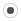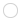## How to load many points in KigEol
Registered MemberPosts
1
Karma
0
OS### How to load many points in Kig

Wed Feb 17, 2010 8:56 pm
Hello.
I have a plain-text file which describes some set of points in the following format:
first line is N - number of points
in second line there are 2*N numbers - X and Y coordinates of each point.

Is there any way to load this file to Kig?
I have tried to write python script for it, but calc() function which I must implement in this script must return only one object (if I'm wrong, please correct me), but I need many objects.
Code: Select all
`def calc(  ):  f = open("input.txt", "r")  n = map(int, f.readline().split())  l = map(int, f.readline().split())  r = []  for i in xrange(0, n):    p = Point(Coordinate(l[2*i], l[2*i+1]))    r.append(p)  return r #Here is error - we cannot return list of objects :(`